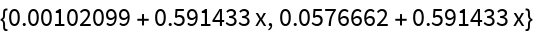#Function Repository Resource:

# MultiNonlinearModelFit

Fit multiple datasets with multiple expressions that share parameters

Contributed by: Sjoerd Smit
 ResourceFunction["MultiNonlinearModelFit"][{dat1,dat2,…},{form1,form2,…},{β1,…},{x1,…}] is a generalized form of NonlinearModelFit where each dataset dati is fitted with expression formi. The expressions are allowed to share parameter values βi with each other. ResourceFunction["MultiNonlinearModelFit"][{dat1,dat2, …}, <|"Expressions"→{form1,form2,…}, "Constraints"→cons |>, {β1,…},{x1,…}] fits the model subject to parameter constraints cons.

## Details and Options

ResourceFunction["MultiNonlinearModelFit"] works by recasting the data and fitting forms into a form suitable for NonlinearModelFit.
The datasets dati must all be numeric matrices.
The number of datasets dati must match the number of expressions formi. It is also possible to specify a function that evaluates to a list when provided with numerical arguments.
The parameters βi can be specified in the same way as in NonlinearModelFit. ResourceFunction["MultiNonlinearModelFit"] also inherits all options from NonlinearModelFit.
Internally, the datasets dati are joined together into a single matrix, so if a Weights vector is specified as an option, it should have the same length as Join[dat1,dat2,]. It is also possible to specify a Weights vector that has a length matching the number of datasets. There is a special option Weights "InverseLengthWeights" to specify that each dataset should weigh equally in the fit, regardless of the number of points in each. Finally, it is also possible to specify the Weights as a list of vectors matching the input data.
The returned model uses the symbol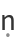(\[FormalN]) to switch between the different expressions formi. This symbol should therefore be avoided by the user when using ResourceFunction["MultiNonlinearModelFit"]. This symbol can be changed with the "DatasetIndexSymbol" option.

## Examples

### Basic Examples

Fit some random data to simple linear models with a shared slope parameter:

 In:=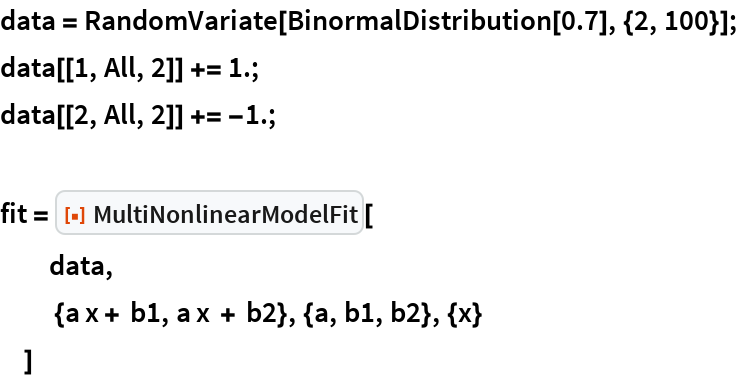Out=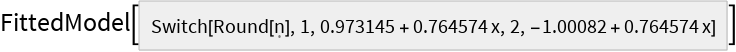In:=Out=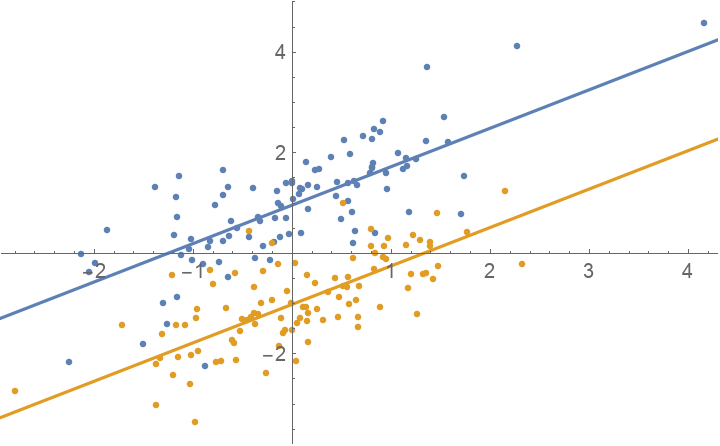Fit the same model with parameter constraints to allow for a small difference between the slopes of the lines. This works best by using a quadratic form for the difference between the slope parameters, since (a1a2)2 is differentiable (unlike Abs[a1a2]):

 In:=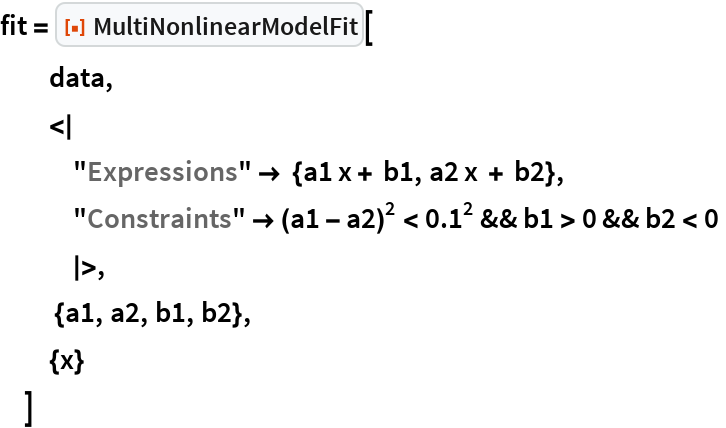Out=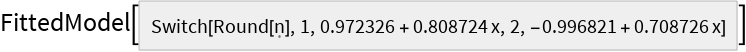In:=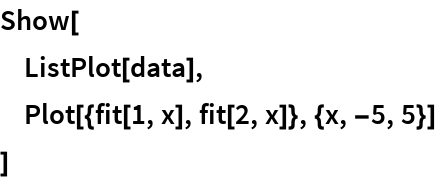Out=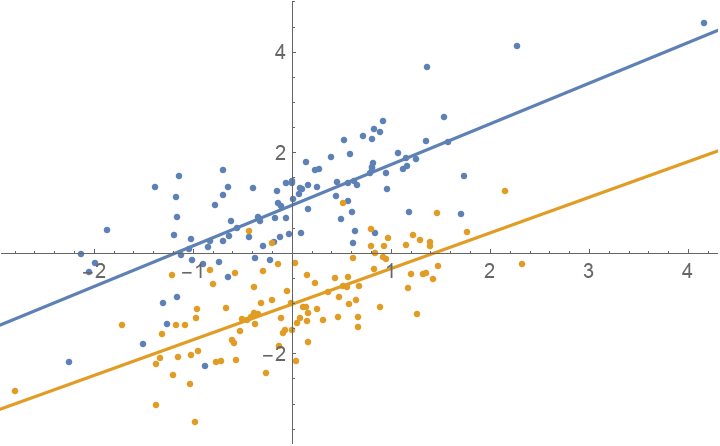### Scope

It is possible to define a fitting function that evaluates to a list of values equal to the number of datasets when provided with numerical parameters: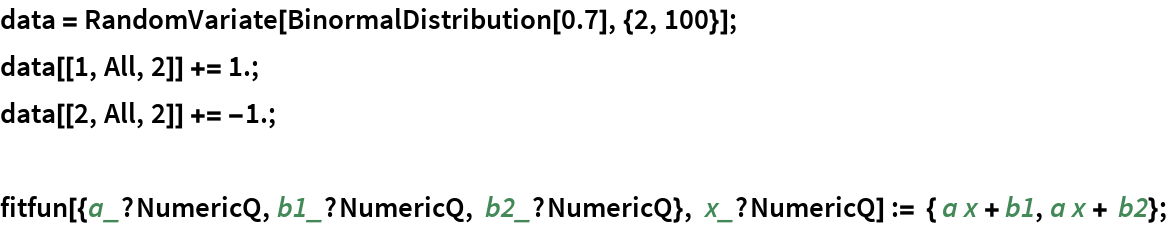The fit function does not evaluate symbolically, only numerically:

 In:=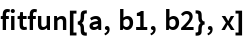Out=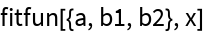In:=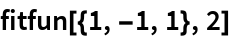Out=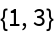Use this function to fit with:

 In:=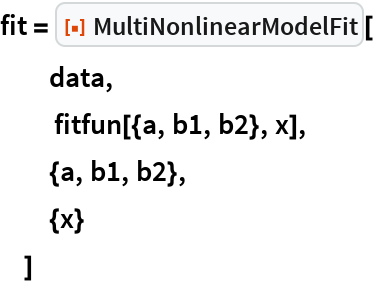Out=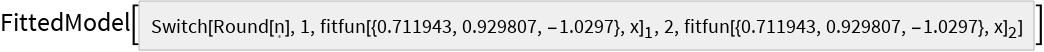In:=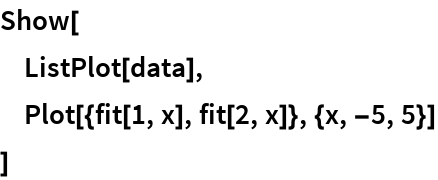Out=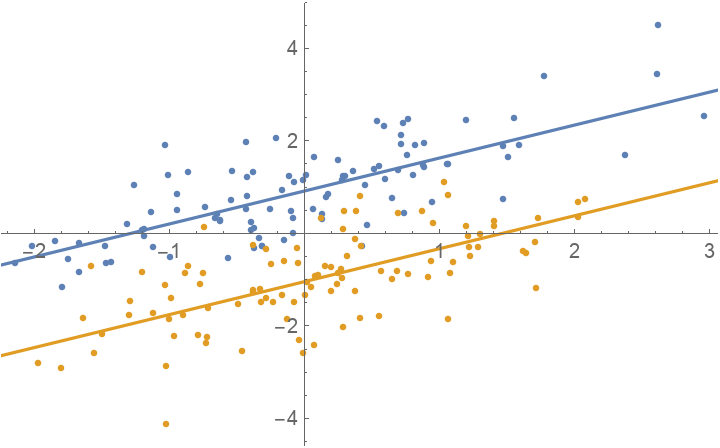Fit two Gaussian peaks with a shared location parameter:

 In:=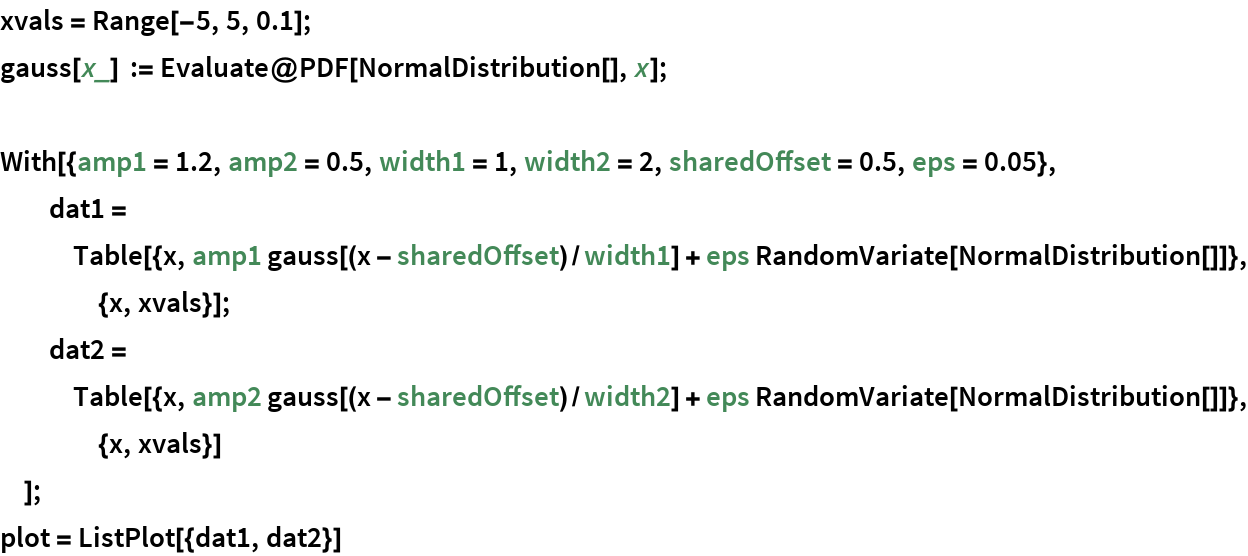Out=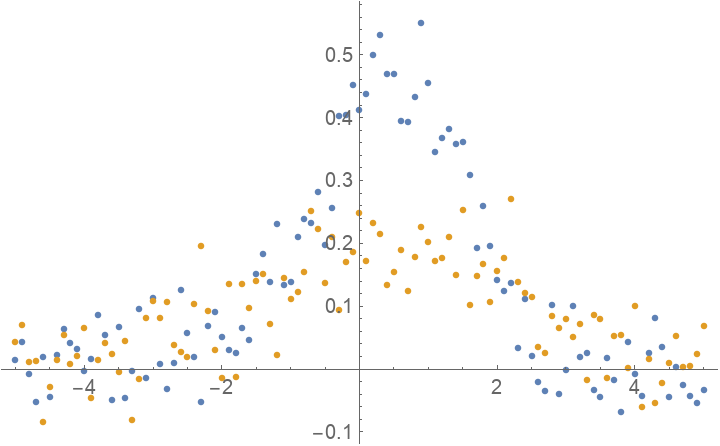Fit with the models that were used to generate the data:

 In:=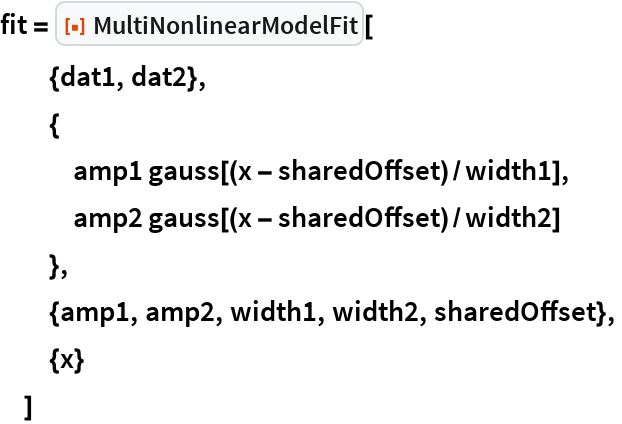Out=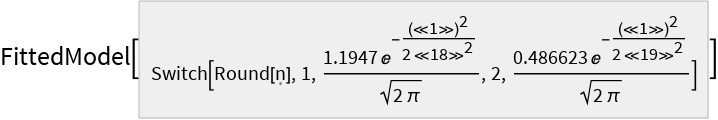In:=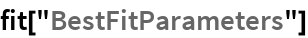Out=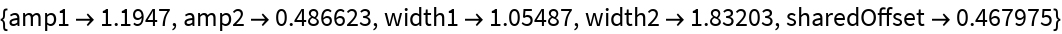Extract the fits as a list of expressions:

 In:=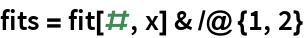Out=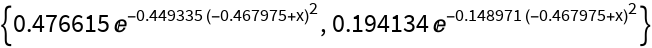Compare the fits to the data:

 In:=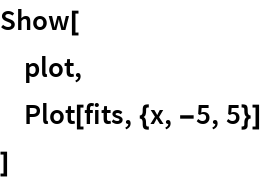Out=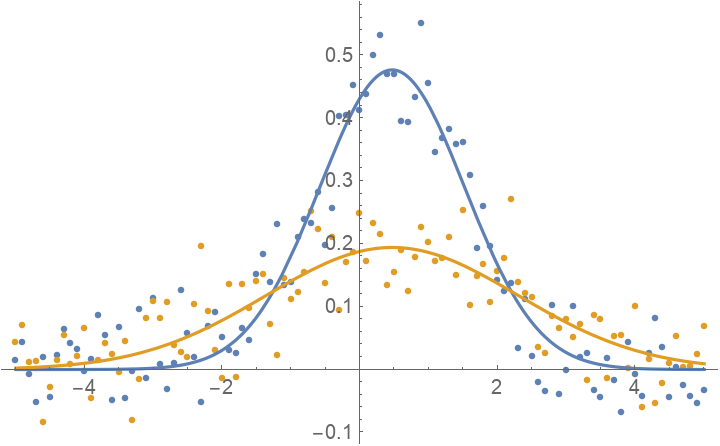### Options

#### Weights

The Weights option can be specified in a number of ways. First generate datasets with an unequal number of points and offset them slightly:

 In:=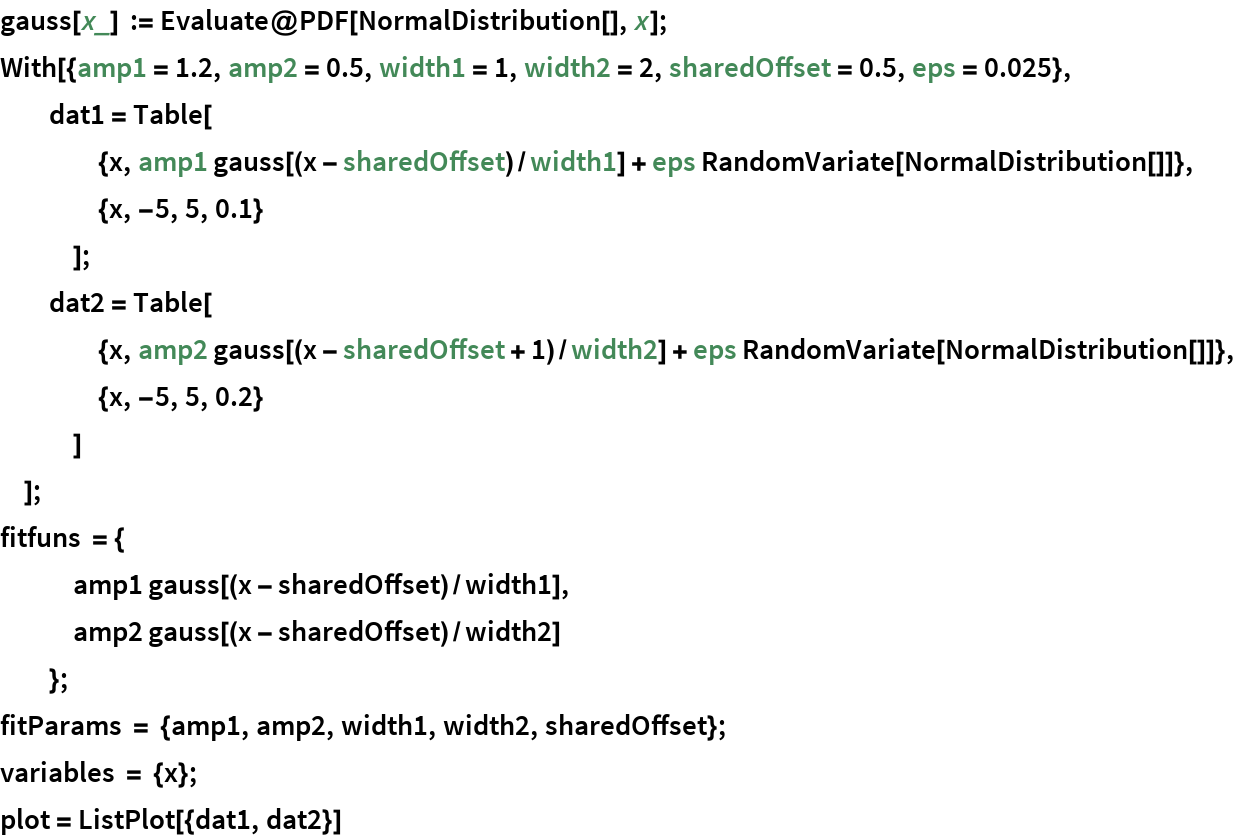Out=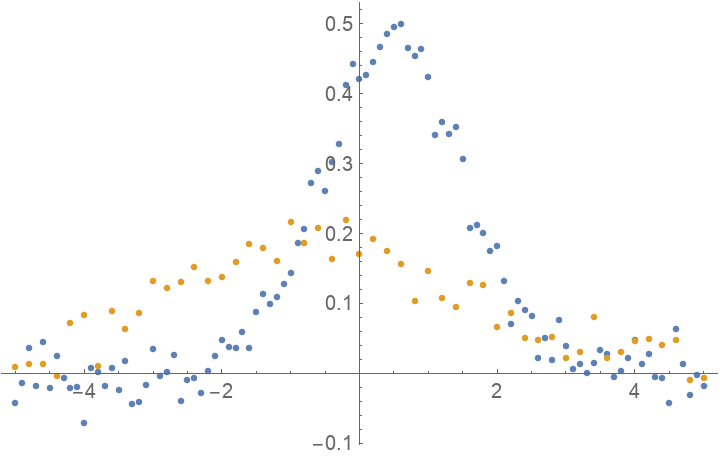Fit the data normally. In this case, each individual data point has equal weights in the fit, so the first dataset gets more weight overall since it has more points:

 In:=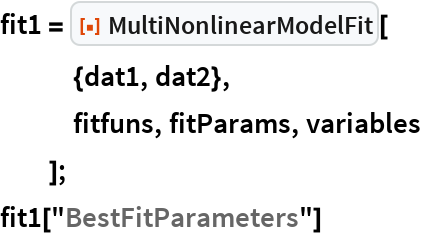Out=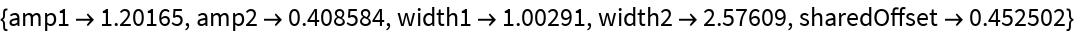In:=Out=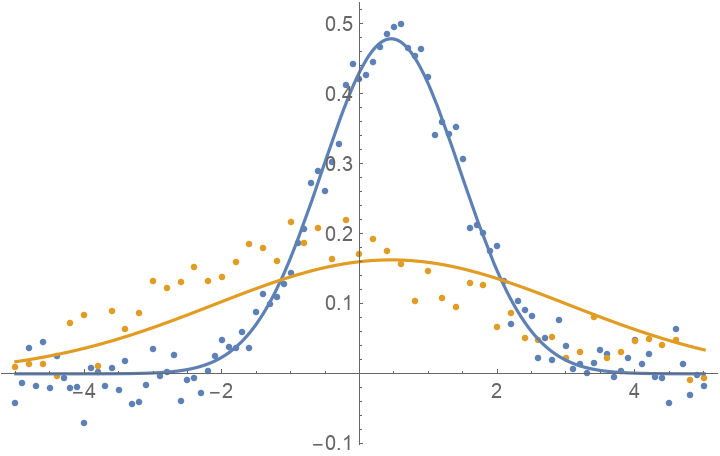Assign more weight to the second dataset:

 In:=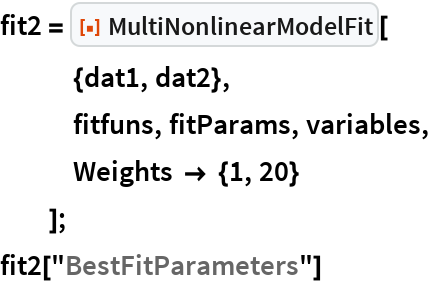Out=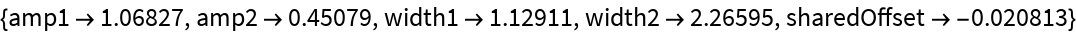In:=Out=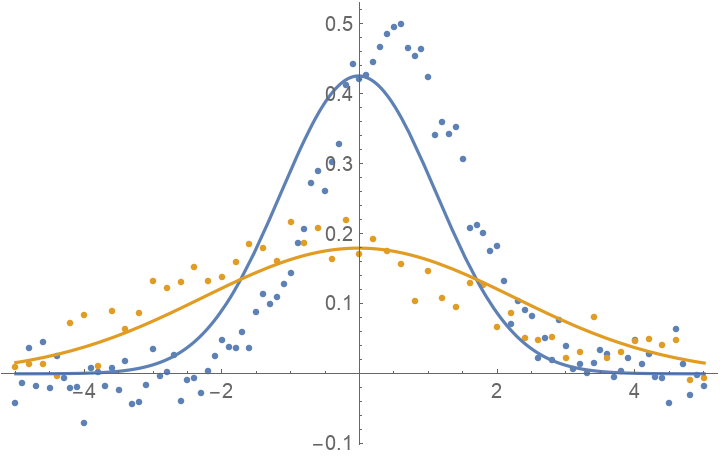Assign weights inversely proportional to the number of points in the dataset. This asserts that each dataset is equally important:

 In:=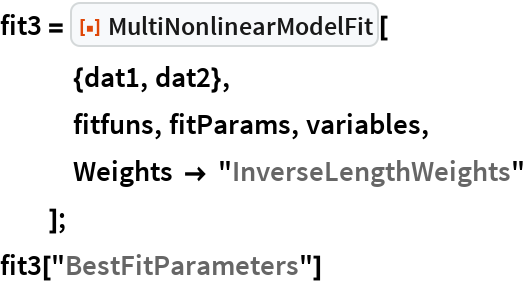Out=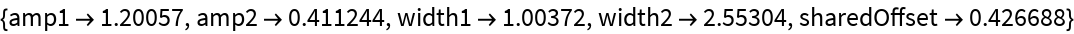In:=Out=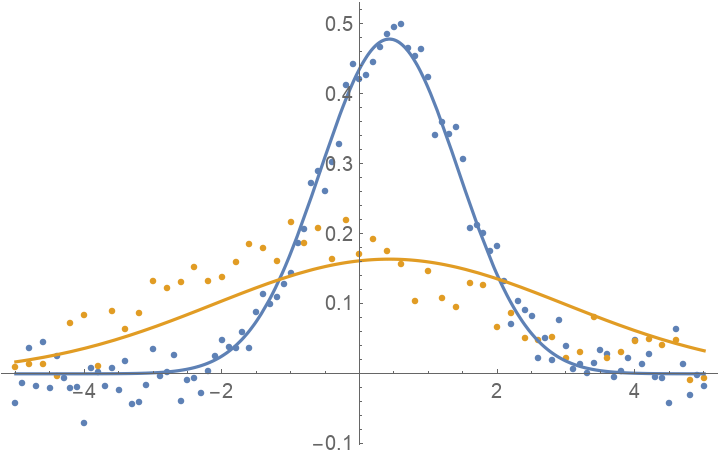To assign weights for each individual data point, you can pass a list of vectors matching the input data:

 In:=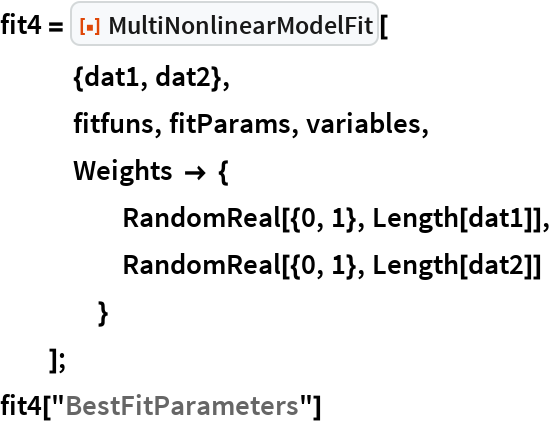Out=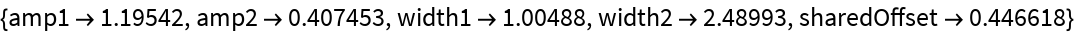In:=Out=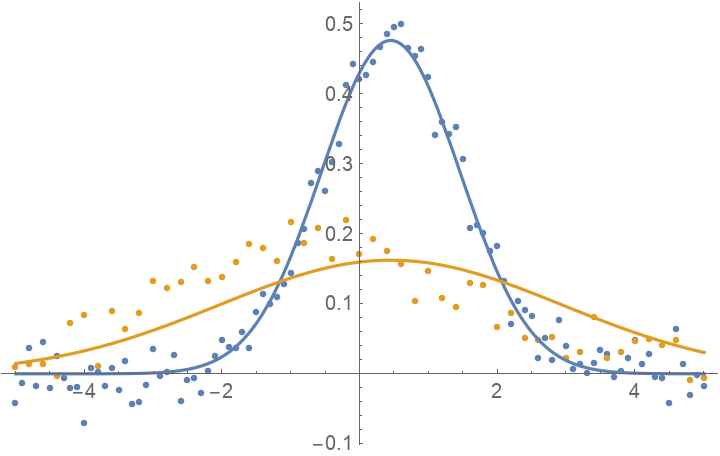#### DatasetIndexSymbol

Use a different symbol to index the datasets:

 In:=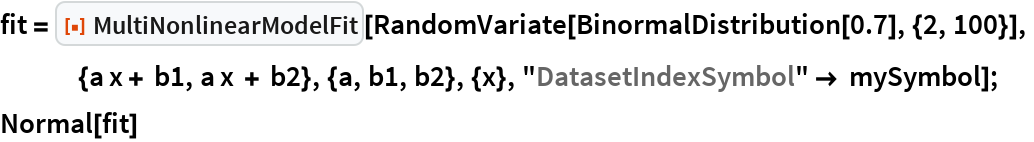Out=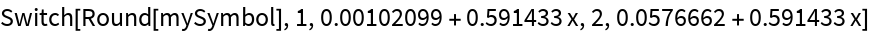In:=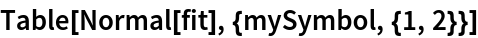Out=